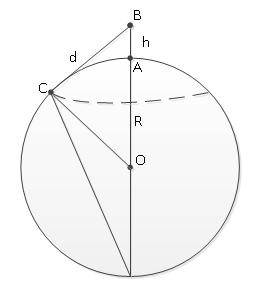# Prospect distance from the ground and line of sight distance between two observers

Calculates the prospect distance from height at observer and line of sight distance between two observers.

The calculator below computes the prospect distance from height at observer and line of sight distance between two observers. Some theory is placed below the calculator.#### Prospect distance from the ground and line of sight distance between two observers

Observer height in meters
Observable object height in meters
Digits after the decimal point: 3
Prospect distance

Line of sight distanceProspect distance
As shown in the picture, the observer's view is limited by the horizon - an imaginary line where the sky touches the earth. According to the picture, we can calculate the distance to the horizon from the Pythagoras theorem.

where R - is Earth radius, usually taken as 6378 kilometers.
Atmospheric refraction increases the prospect distance by over 6 percent.
The result formula is

Simplified formula, which can be deduced from above, is often used
, for nautical miles or
, for kilometersLine of sight
As shown in the picture, line of sight distance is simply an addition of two prospect distances.

P.S. The article, as you may have noticed, is based on the assumption that the Earth is spherical. Usually, flat-earth supporters run into such articles and begin to refute the calculation based on personal experience. It's always hilarious

URL copied to clipboardPLANETCALC, Prospect distance from the ground and line of sight distance between two observers